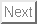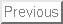# Abstracts of papers

On asynchrony in name-passing calculi

The asynchronous pi-calculus is considered the basis of experimental programming languages (or proposal of programming languages) like Pict, Join, and Blue calculus. However, at a closer inspection, these languages are based on an even simpler calculus, called piA, where: (a) only the output capability of names may be transmitted; (b) there is no matching or similar constructs for testing equality between names.

We study the basic operational and algebraic theory of piA. We focus on bisimulation-based behavioural equivalences, precisely on barbed congruence . We prove two coinductive characterisations of barbed congruence in piA, and some basic algebraic laws. We then show applications of this theory, including: the derivability of delayed input ; the correctness of an optimisation of the encoding of call-by-name lambda-calculus; the validity of some laws for Join.

Imperative Objects and Mobile Processes

An interpretation of Abadi and Cardelli's first-order Imperative Object Calculus into a typed pi-calculus is presented. The interpretation validates the subtyping relation and the typing judgements of the Object Calculus, and is computationally adequate. The proof of computational adequacy makes use of (a pi-calculus version) of ready simulation , and of a factorisation of the interpretation into a functional part and a very simple imperative part.

The interpretation can be used to compare and contrast the Imperative and the Functional Object Calculi, and to prove properties about them, within a unified framework.

Typed pi-calculus at work: a correctness proof of Jones's parallelisation transformation on concurrent objects

Cliff Jones has proposed transformations between concrete programs and general transformation rules that increase concurrency in a system of objects, and has raised the challenge of how to prove their validity. We present a proof of correctness of the hardest of Jones's concrete transformations. The proof uses a typed pi-calculus and typed behavioural equivalences. Our type system tracks receptiveness; it guarantees that the input-end of certain channels is always ready to receive messages (at least as long as there are processes that could send such messages), and that all messages will be processed using the same continuation. This work is also intended as an example of the usefulness of pi-calculus types for reasoning.

The name discipline of uniform receptiveness

In a process calculus, we say that a name x is uniformly receptive for a process P if: (1) at any time P is ready to accept an input at x, at least as long as there are processes that could send messages at x; (2) the input offer at x is functional, that is, all messages received by P at x are applied to the same continuation. In the pi-calculus this discipline is employed, for instance, when modeling functions, objects, higher-order communications, remote-procedure calls. We formulate the discipline of uniform receptiveness by means of a type system, and then we study its impact on behavioural equivalences and process reasoning. We develop some theory and proof techniques for uniform receptiveness, and illustrate their usefulness on some non-trivial examples.

Behavioral Equivalence in the Polymorphic Pi-Calculus

We investigate parametric polymorphism in message-based concurrent programs, focusing on behavioral equivalences in a typed process calculus analogous to the polymorphic lambda-calculus of Girard and Reynolds.

Polymorphism constrains the power of observers by preventing them from directly manipulating data values whose types are abstract, leading to notions of equivalence much coarser than the standard untyped ones. We study the nature of these constraints through simple examples of concurrent abstract data types and develop basic theoretical machinery for establishing bisimilarity of polymorphic processes.

We also observe some surprising interactions between polymorphism and aliasing, drawing examples from both the polymorphic pi-calculus and ML.

On Bisimulations for the Asynchronous pi-calculus

The asynchronous pi-calculus is a variant of the pi-calculus where message emission is non-blocking. Honda and Tokoro have studied a semantics for this calculus based on bisimulation. Their bisimulation relies on a modified transition system where, at any moment, a process can perform any input action.

In this paper we propose a new notion of bisimulation for the asynchronous pi-calculus, defined on top of the standard labelled transition system. We give several characterizations of this equivalence including one in terms of Honda and Tokoro's bisimulation, and one in terms of barbed equivalence. We show that this bisimulation is preserved by name substitutions, hence by input prefix. Finally, we give a complete axiomatization of the (strong) bisimulation for finite terms.

A Fully-Abstract Model for the pi-calculus

This paper provides both a fully abstract (domain-theoretic) model for the pi-calculus and a universal (set-theoretic) model for the finite pi-calculus with respect to strong late bisimulation and congruence. This is done by: considering categorical models, defining a metalanguage for these models, and translating the pi-calculus into the metalanguage.

A technical novelty of our approach is an abstract proof of full abstraction: The result on full abstraction for the finite pi-calculus in the set-theoretic model is axiomatically extended to the whole pi-calculus with respect to the domain-theoretic interpretation. In this proof, a central role is played by the description of non-determinism as a free construction and by the equational theory of the metalanguage.

A Partition Refinement Algorithm for the pi-calculus

The partition refinement algorithm is the basis for most of the tools for checking bisimulation equivalences and for computing minimal realisations of CCS-like finite state processes.

In this paper, we present a partition refinement algorithm for the pi-calculus, a development of CCS where channel names can be communicated. It can be used to check bisimilarity and to compute minimal realisations of finite control processes -- the pi-calculus counterpart of CCS finite state processes. The algorithm is developed for strong open bisimulation, and can be adapted to late and early bisimulations, as well as to weak bisimulations. To arrive at the algorithm, a few laws, proof techniques, and four characterizations of open bisimulation are proved.

An interpretation of Typed Objects into Typed pi-calculus

An interpretation of Abadi and Cardelli's first-order functional Object Calculus into a typed pi-calculus is presented. The interpretation validates the subtyping relation and the typing judgements of the Object Calculus, and is computationally adequate. This is the first interpretation of a typed Object-Oriented language into a process calculus. The study intends to offer a contribution to understanding on the one hand, the relationship between pi-calculus types and conventional types of programming languages and on the other hand, the usefulness of the pi-calculus as a metalanguage for the semantics of typed Object-Oriented languages.

The type language for the pi-calculus has Pierce and Sangiorgi's I/O annotations, to separate the capabilities of reading and writing on a channel, and variant types. Technical contributions of the paper are the presentation of variant types for the pi-calculus and their typing and subtying properties, and an analysis of behavioural equivalences in a pi-calculus with variant types.

Some Congruence Properties for pi-calculus Bisimilarities

Both for interleaving and for non-interleaving semantics, several variants of a pi-calculus bisimilarity can be given which differ on the requirements imposed on name instantiations. Examples are the late, early, open and ground variants. The ground variant is the simplest because it places no requirements on name instantiations. With the exception of open bisimilarities, none of the bisimilarity considered in the literature is a congruence relation on the full pi-calculus language.

We show that in the case of (certain forms of) causal bisimulation the late, early, open and ground variants coincide and are congruence relations in the sublanguage of the pi-calculus without matching. We also show that to obtain the same results in the case of the interleaving bisimilarity, in addition to forbidding matching it is necessary to constrain the output prefix.

On the bisimulation proof method

The most popular method for establishing bisimilarities among processes is to exhibit bisimulation relations. By definition, R is a bisimulation relation if R progresses to R itself, i.e., pairs of processes in R can match each other's actions and their derivatives are again in R.

We study generalisations of the method aimed at reducing the size of the relations to exhibit and hence relieving the proof work needed to establish bisimilarity results. We allow a relation R to progress to a different relation F (R), where F is a function on relations. Functions which can be safely used in this way (i.e., such that if R progresses to F( R), then R only includes pairs of bisimilar processes) are sound. We give a simple condition which ensures soundness. We show that the class of sound functions contains non-trivial functions and we study the closure properties of the class with respect to various important function constructors, like composition, union and iteration. These properties allow us to construct sophisticated sound functions--and hence sophisticated proof techniques for bisimilarity--from simpler ones.

The usefulness of our proof techniques is supported by various non-trivial examples drawn from the process algebras CCS and pi-calculus . They include the proof of the unique solution of equations and the proof of a few properties of the replication operator. Among these, there is a novel result which justifies the adoption of a simple form of prefix-guarded replication as the only form of replication in the pi-calculus .

pi-calculus, internal mobility and agent-passing calculi

The pi-calculus is a process algebra which originates from CCS and permits a natural modelling of mobility (i.e., dynamic reconfigurations of the process linkage) using communication of names. Previous research has shown that the pi-calculus has much greater expressiveness than CCS, but also a much more complex mathematical theory. The primary goal of this work is to understand the reasons of this gap. Another goal is to compare the expressiveness of name-passing calculi, i.e., calculi like pi-calculus where mobility is achieved via exchange of names, and that of agent-passing calculi, i.e., calculi where mobility is achieved via exchange of agents.

We separate the mobility mechanisms of the pi-calculus into two, respectively called internal mobility and external mobility. The study of the subcalculus which only uses internal mobility, called pi-I, suggests that internal mobility is responsible for much of the expressiveness of the pi-calculus , whereas external mobility is responsible for many of the semantic complications. A pleasant property of pi-I is the full symmetry between input and output constructs.

Internal mobility is strongly related to agent-passing mobility. By imposing bounds on the order of the types of pi-I and of the Higher-Order pi-calculus we define a hierarchy of name-passing calculi based on internal mobility and one of agent-passing calculi. We show that there is an exact correspondence, in terms of expressiveness, between the two hierarchies.

Lazy functions and mobile processes

This paper continues the study of Milner's encoding of the lazy lambda-calculus into the pi-calculus. The encoding is shown to give rise to a lambda-model in which, in accordance with the theory of the lazy lambda-calculus, conditional extensionality holds. However, the model is not fully abstract. To obtain full abstraction, the operational equivalence on lambda-terms (applicative bisimulation) is refined. The resulting relation (open applicative bisimulation) allows us to observe some internal structure of lambda-terms, and coincides with the Lévy-Longo Tree equality.

Milner's encoding is presented on a sublanguage of the pi-calculus similar to those proposed by Honda and Tokoro, and Boudol. Some properties of bisimulation on this sublanguage are demonstrated and used to simplify a few proofs in the paper. For instance, ground bisimulation, a form of bisimulation where no name instantiation on input actions is required, is proved to be a congruence relation; as a corollary, various pi-calculus bisimilarities (ground, late, early, open) are shown to coincide on this sublanguage.

Bisimulation in higher-order calculi

A higher-order process calculus is a calculus for communicating systems which contains higher-order constructs like communication of terms. We analyse the notion of bisimulation in these calculi. We argue that both the standard definition of bisimulation (i.e., the one for CCS and related calculi), as well as higher-order bisimulation (studied by Astesiano-Giovini-Reggio, Boudol, and Thomsen) are in general unsatisfactory, because over-discriminating.

We propose and study a new form of bisimulation for such calculi, called context bisimulation, which yields a more satisfactory discriminanting power. A drawback of context bisimulation is the heavy use of universal quantification in its definition. A major goal of the paper is to find characterisations which make bisimilarities easier to verify.

An important role in our theory is played by the factorisation theorem: When comparing the behaviour of two processes, it allows us to isolate'' subcomponents which might cause differences, so that the analysis can be concentrated on them.

Techniques of weak bisimulation up-to

Bisimulation up to'' is a technique for reducing the size of the relation needed to define a bisimulation. It works smoothly in the strong case, where it was first introduced by Milner. But this does not directly generalize to the weak case, as erroneously reported in Milner's book. To overcome this problem, two new up-to'' techniques are proposed: They are respectively based on the use of expansion and of almost-weak bisimulation. The second solution is more general than the first one, but expansion enjoys a nicer mathematical treatment. The usefulness and generality of the solutions is motivated with non-trivial examples: two different implementations of a sorting machine.

A fully abstract semantics for causality in the pi-calculus

We examine the meaning of causality in calculi for mobile processes like the pi-calculus , and we investigate the relationship between interleaving and causal semantics for such calculi.

We separate two forms of causal dependencies on actions of pi-calculus processes, called subject and object dependencies: The former originate from the nesting of prefixes and are propagated through interactions among processes (they are the only form of causal dependencies present in CCS-like languages); the latter originate from the binding mechanisms on names. We propose a notion of causal bisimulation which distinguishes processes which differ for the subject or for the object dependencies. We show that this causal equivalence can be reconducted to, or implemented into, the ordinary interleaving observation equivalence. We prove that our encoding is fully abstract w.r.t. the two behavioural equivalences. This allows us to exploit the simpler theory of the interleaving semantics to reason about the causal one.

In a previous paper, we have carried out a similar programme is for location bisimulation, a non-interleaving spatial-sensitive (as opposed to causal-sensitive) behavioural equivalence. The comparison between the encodings of causal bisimulation, and of location bisimulation, evidences the similarities and the differences between these two equivalences.

Localities and true-concurrency in calculi for mobile processes

Process algebra semantics can be categorised into non-interleaving semantics, where parallel composition is considered a primitive operator, and interleaving semantics, where concurrency is reduced to sequentiality plus nondeterminism. The former have an appealing intuitive justification, but the latter are mathematically more tractable.

This paper addresses the study of non-interleaving semantics in the framework of process algebras for mobile systems, like pi-calculus . We focus on location bisimulation (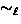), in our opinion one of the most convincing non-interleaving equivalences, which aims to describe the spatial dependencies on processes. We introducein pi-calculus following the definition for CCS given Boudol, Castellani, Hennessy and Kiehn.. Our main contribution is to show that in pi-calculuscan be expressed, or implemented, within the ordinary interleaving observation equivalence by means of a fairly simple and fully abstract encoding. Thus, we can take advantage of the easier theory of observation equivalence to reason about. We illustrate this with a few examples, including the proof of the congruence properties of. We show that in pi-calculusis not a congruence, and that the full abstraction of the encoding extends to the induced congruence.

The results in the paper also shed more light on the expressive power of the pi-calculus .

A theory of bisimulation for pi-calculus

We study a new formulation of bisimulation for the pi-calculus , which we have called open bisimulation (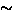). In contrast with the previously known bisimilarity equivalences,is preserved by all pi-calculus operators, including input prefix. The differences among all these equivalences already appear in the sublanguage without name restrictions: Here the definition ofcan be factorised into a standard'' part which, modulo the different syntax of actions, is the CCS bisimulation, and a part specific to the pi-calculus , which requires name instantiation. Attractive features ofare: A simple axiomatisation (of the finite terms), with a completeness proof which leads to the construction of minimal canonical representatives for the equivalence classes of; an efficient'' characterisation, based on a modified transition system. This characterisation seems promising for the development of automated-verification tools and also shows the call-by-need flavour of. Although in the paper we stick to the pi-calculus , the issues developed may be relevant to value-passing calculi in general.

Typing and subtyping for mobile processes

The pi-calculus is a process algebra that supports mobility by focusing on the communication of channels. Milner's presentation of the pi-calculus includes a type system assigning arities to channels and enforcing a corresponding discipline in their use. We extend Milner's language of types by distinguishing between the ability to read from a channel, the ability to write to a channel, and the ability both to read and to write. This refinement gives rise to a natural subtype relation similar to those studied in typed lambda-calculi. The greater precision of our type discipline yields stronger versions of standard theorems about the pi-calculus . These can be used, for example, to obtain the validity of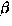-reduction for the more efficient of Milner's encodings of the call-by-value lambda-calculus, which fails in the ordinary pi-calculus . We define the syntax, typing, subtyping, and operational semantics of our calculus, prove that the typing rules are sound, apply the system to Milner's lambda-calculus encodings, and sketch extensions to higher-order process calculi and polymorphic typing.

An investigation into functions as processes   Milner has examined the encoding of the lambda-calculus into the pi-calculus. The former is the universally accepted basis for computations with functions, the latter aims at being its counterpart for computations with processes. The primary goal of this paper is to continue the study of Milner's encodings. We focus mainly on the lazy lambda-calculus. We show that its encoding gives rise to a lambda-model, in which a weak form of extensionality holds. However the model is not fully abstract: To obtain full abstraction, we examine both the restrictive approach, in which the semantic domain of processes is cut down, and the expansive approach, in which lambda-calculus is enriched with constants to obtain a direct characterisation of the equivalence on lambda-terms induced, via the encoding, by the behavioural equivalence adopted on the processes. Our results are derived exploiting an intermediate representation of Milner's encodings into the Higher-Order pi-calculus, an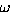-order extension of pi-calculus where also agents may be transmitted. For this, essential use is made of the fully abstract compilation from the Higher-Order pi-calculus to the pi-calculus studied in Sangiorgi's thesis.

From pi-calculus to Higher-order pi-calculus -- and back

We compare the first-order and the higher-order paradigms for the representation of mobility in process algebras. The prototypical calculus in the first-order paradigm is the pi-calculus . By generalising its sort mechanism we derive an-order extension, called Higher-Order pi-calculus (HOpi). We give examples of its use, including the encoding of lambda-calculus. Surprisingly, we show that such an extension does not add expressiveness: Higher-order processes can be faithfully represented at first order. We conclude that the first-order paradigm, which enjoys a simpler and more intuitive theory, should be taken as basic. Nevertheless, the study of the lambda-calculus encodings shows that a higher-order calculus can be very useful for reasoning at a more abstract level.

Barbed Bisimulation   We propose barbed bisimulation as a tool to describe bisimulation-based equivalence uniformly for any calculi possessing (a) a reduction relation and (b) a convergency predicate which simply detects the possibility of performing some observable action. This opens interesting perspectives for the adoption of a reduction semantics in process algebras. As a test-case we prove that strong bisimulation of CCS coincides with the congruence induced by barbed bisimulation.

Algebraic theories for name-passing calculi

In a theory of processes the names are atomic data items which can be exchanged and tested for identity. A well-known example of a calculus for name-passing is the pi-calculus, where names additionally are used as communication ports. We provide complete axiomatisations of late and early bisimulation equivalences in such calculi. Since neither of the equivalences is a congruence we also axiomatise the corresponding largest congruences. We consider a few variations of the signature of the language; among these, a calculus of deterministic processes which is reminiscent of sequential functional programs with a conditional construct. Most of our axioms are shown to be independent. The axiom systems differ only by a few simple axioms and reveal the similarities and the symmetries of the calculi and the equivalences.

The Lazy Lambda Calculus in a Concurrency Scenario

The use of lambda-calculus in richer settings, possibly involving parallelism, is examined in terms of the effect on the equivalence between lambda-terms. We concentrate on Abramsky's lazy lambda-calculus and we follow two directions. Firstly, the lambda-calculus is studied within a process calculus by examining the equivalenceinduced by Milner's encoding into the pi-calculus. We start from a characterisation ofpresented in Sangiorgi's thesis. We derive a few simpler operational characterisations, from which we prove full abstraction w.r.t. Levy-Longo Trees. Secondly, we examine Abramsky's applicative bisimulation when the lambda-calculus is augmented with (well-formed) operators, that is symbols equipped with reduction rules describing their behaviour. In this way, the maximal discrimination between pure lambda-terms (i.e., the finest behavioural equivalence) is obtained when all operators are used. We prove that the presence of certain non-deterministic operators is sufficient and necessary to induce it and that it coincides with the discrimination given by.We conclude that the introduction of non-determinism into the lambda-calculus is exactly what makes applicative bisimulation appropriate for reasoning about the functional terms when concurrent features are also present in the language, or when they are embedded into a concurrent language.

An Improved Systolic Array for String Correction

The theory of systolic automata has served in the past for comparative assessments of the computational power of different systolic arrays. Here, it is used in the design of a systolic array. Theorems from the theory of systolic automata guide the transformational improvement of a known systolic array for string correction.Davide Sangiorgi
2/13/1998